SBOS748 September   2016

PRODUCTION DATA.

1. Features
2. Applications
3. Description
1.     Device Images
4. Revision History
5. Device Comparison Table
6. Pin Configuration and Functions
7. Specifications
8. Parameter Measurement Information
9. Detailed Description
1. 9.1 Overview
2. 9.2 Functional Block Diagram
3. 9.3 Feature Description
4. 9.4 Device Functional Modes
10. 10Applications and Implementation
1. 10.1 Application Information
2. 10.2 Typical Application
1. 10.2.1 Design Requirements
2. 10.2.2 Detailed Design Procedure
3. 10.2.3 Application Curves
11. 11Power Supply Recommendations
12. 12Layout
13. 13Device and Documentation Support
14. 14Mechanical, Packaging, and Orderable Information

• DGK|8
• DGK|8

### 7.6 Typical Characteristics

at TA = 25°C, IL = 0 mA, and VIN = 5 V, using REF6225 (unless otherwise noted)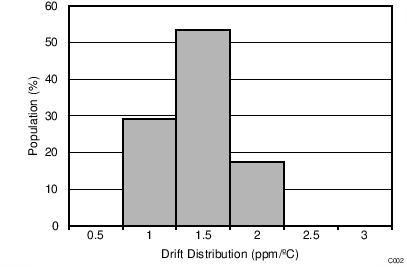TA = 0°C to +70°C
Figure 1. Drift Distribution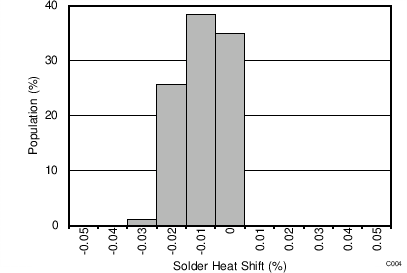Figure 3. Solder-Heat Shift Distribution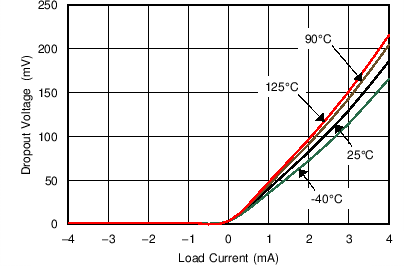Figure 5. Dropout Voltage vs Load Current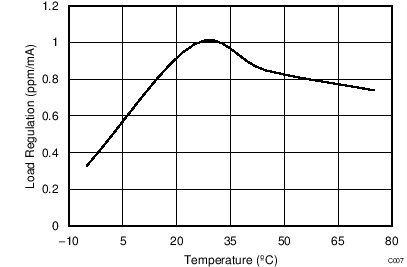VIN = VOUT + 600 mV, IL = 0 mA to 4 mA
Figure 7. Load Regulation Sinking vs TemperatureFigure 9. Supply Current vs TemperatureFigure 11. Turn-On Settling Time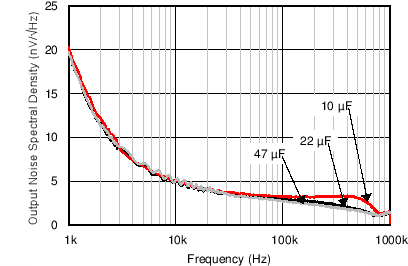Figure 13. Output-Voltage Noise Spectrum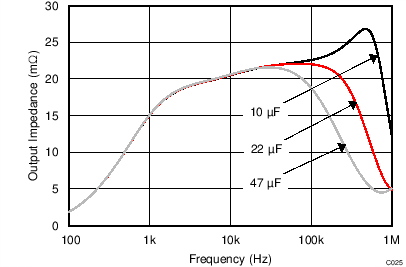Graph obtained by design simulation
Figure 15. Output Impedance vs Frequency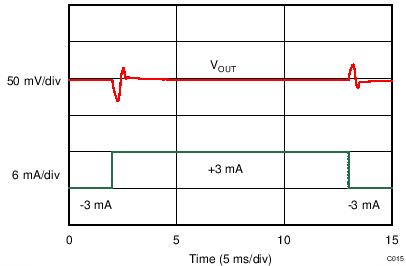Load current = ±3 mA
Figure 17. Load Transient Response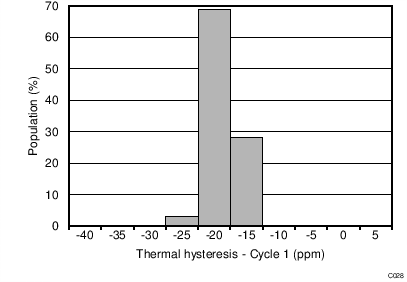Figure 19. Thermal Hysteresis Distribution (Cycle 1)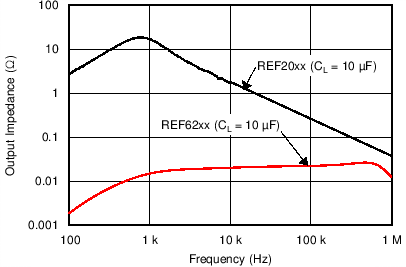Figure 21. Output Impedance ComparisonREF6250 driving REF pin of ADS8881, fIN = 2 kHz, SNR = 100.4 dB, THD = –123.9 dB
Figure 23. Typical FFT Plot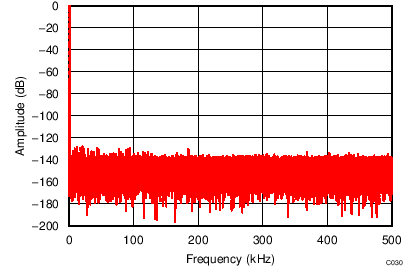REF6241 driving REF pin of ADS8881, fIN = 1 kHz, SNR = 99 dB, THD = –124.4 dB
Figure 25. Typical FFT Plot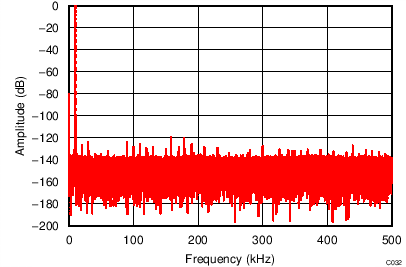REF6241 driving REF pin of ADS8881, fIN = 10 kHz, SNR = 97.2 dB, THD = –119.7 dB
Figure 27. Typical FFT Plot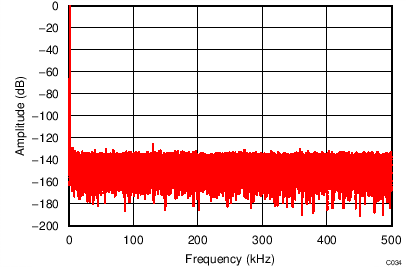REF6225 driving REF pin of ADS8881, fIN = 2 kHz, SNR = 95.4 dB, THD = –123.5 dB
Figure 29. Typical FFT Plot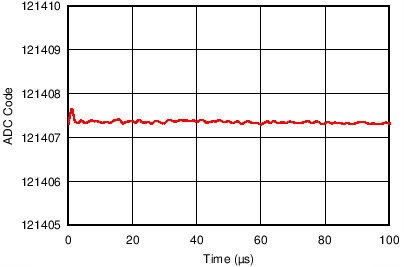REF6250 driving REF pin of ADS8881 operating at 1 MSPS, positive full-scale input to ADS8881
Figure 31. Reference Droop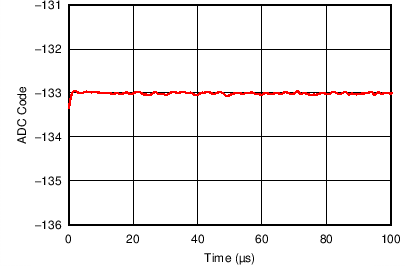REF6250 driving REF pin of ADS8881 operating at 1 MSPS, AINP = AINN = VREF / 2 for ADS8881
Figure 33. Reference Droop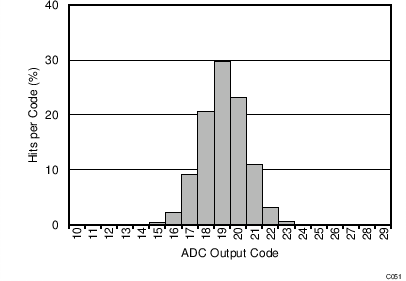AINP = AINN = VREF / 2 for ADS8881, sampling rate = 500 kSPS
Figure 35. DC Input Histogram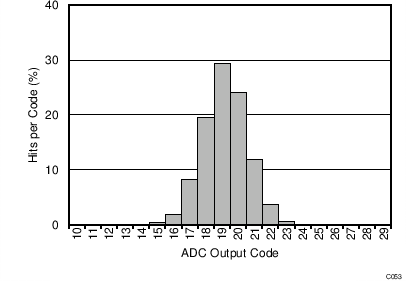AINP = AINN = VREF / 2 for ADS8881, sampling rate = 20 kSPS
Figure 37. DC Input Histogram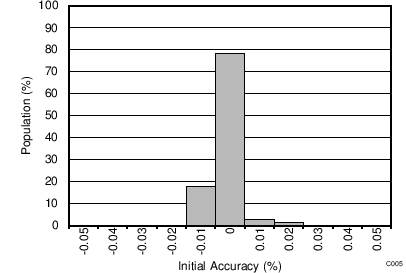Figure 2. Initial Accuracy DistributionFigure 4. Output Voltage Accuracy vs Temperature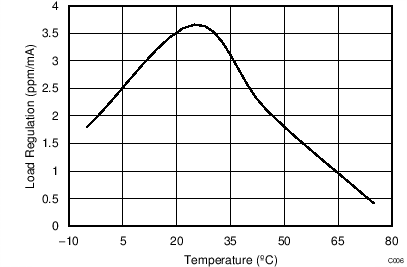VIN = VOUT + 600 mV, IL = 0 mA to 4 mA
Figure 6. Load Regulation Sourcing vs Temperature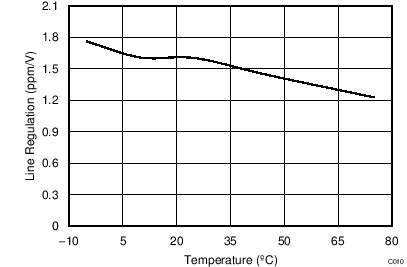VOUT + 0.25 V ≤ VIN ≤ 5.5 V
Figure 8. Line Regulation vs Temperature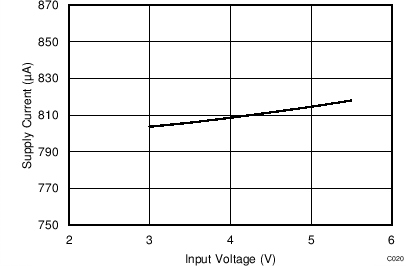Figure 10. Supply Current vs Input Voltage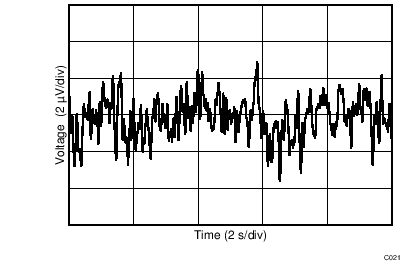Figure 12. 0.1-Hz to 10-Hz Noise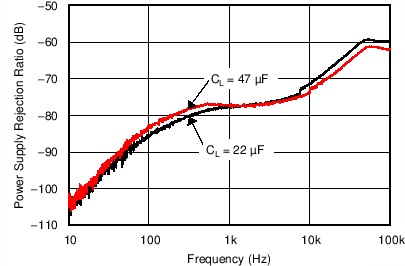Figure 14. PSRR vs FrequencyLoad current = ±1 mA
Figure 16. Load Transient Response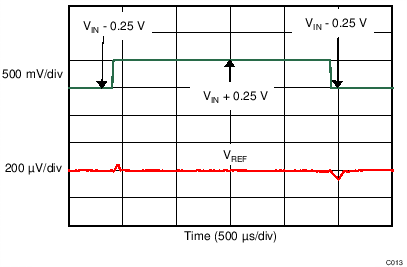Figure 18. Line Transient Response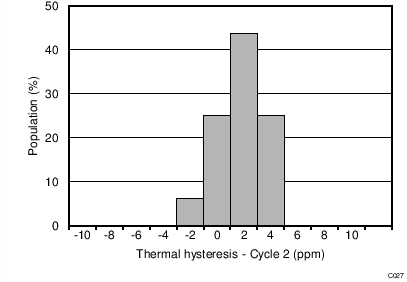Figure 20. Thermal Hysteresis Distribution (Cycle 2)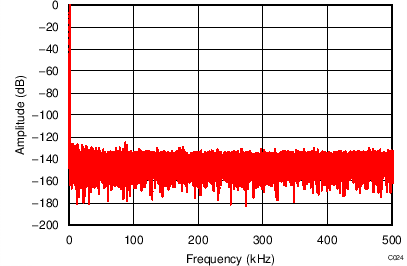REF6250 driving REF pin of ADS8881, fIN = 1 kHz, SNR = 100.5 dB, THD = –125.9 dB
Figure 22. Typical FFT Plot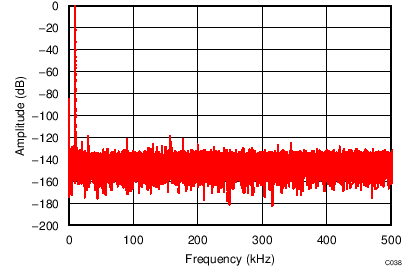REF6250 driving REF pin of ADS8881, fIN = 10 kHz, SNR = 99.2 dB, THD = –119.4 dB
Figure 24. Typical FFT Plot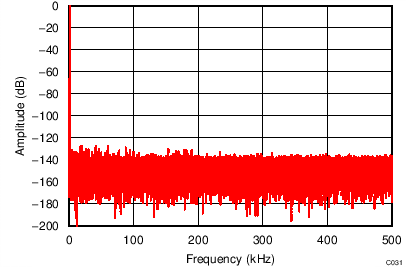REF6241 driving REF pin of ADS8881, fIN = 2 kHz, SNR = 99 dB, THD = –123.6 dB
Figure 26. Typical FFT Plot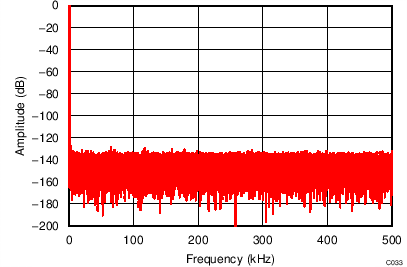REF6225 driving REF pin of ADS8881, fIN = 1 kHz, SNR = 95.4 dB, THD = –124 dB
Figure 28. Typical FFT Plot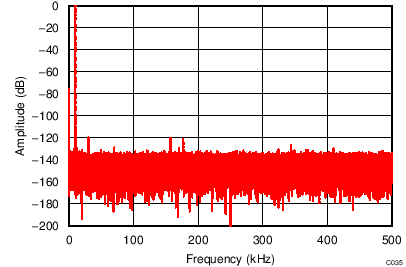REF6225 driving REF pin of ADS8881, fIN = 10 kHz, SNR = 94.0 dB, THD = –119.3 dB
Figure 30. Typical FFT Plot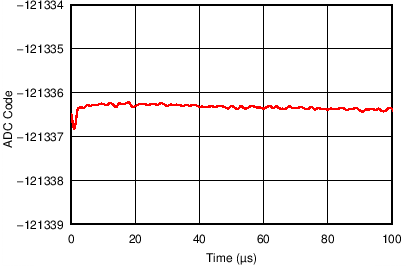REF6250 driving REF pin of ADS8881 operating at 1 MSPS, negative full-scale input to ADS8881
Figure 32. Reference Droop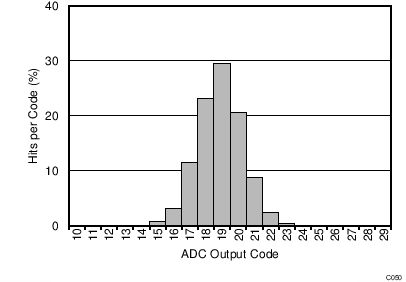AINP = AINN = VREF / 2 for ADS8881, sampling rate = 1 MSPS
Figure 34. DC Input Histogram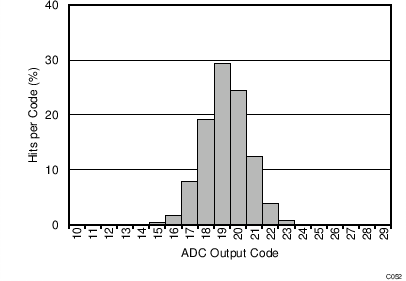AINP = AINN = VREF / 2 for ADS8881, sampling rate = 100 kSPS
Figure 36. DC Input Histogram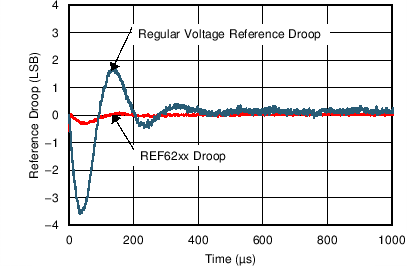1 LSB = 19.07 µV, with ADS8881 at 1 MSPS
Figure 38. Reference Droop Comparison